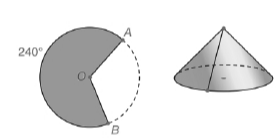Chapter 9.3, Problem 48E### Elementary Geometry for College St...

6th Edition
Daniel C. Alexander + 1 other
ISBN: 9781285195698

#### Solutions

Chapter
Section### Elementary Geometry for College St...

6th Edition
Daniel C. Alexander + 1 other
ISBN: 9781285195698
Textbook Problem
1 views

# When radii O A ¯ and O B ¯ are placed so that they coincide, a 240 ∘ sector of a circle is sealed to form a right circular cone. If the length of the radius of the circle is 6.4 cm, what is the length of the approximate lateral area of the cone that is formed? Use a calculator and round the answer to the nearest tenth of a square inch.To determine

To find:

The approximate lateral area of the cone that is formed.

Explanation

Given:

When radii OA and OB are placed so that they coincide, a 240 sector of a circle is sealed to form a right circular cone. The radius of the circle is 6.4 cm.

Calculation:

In a circle of radius r, the area of the sector whose arc measure is m is A=m360πr2.

We know 1 inch=2.54 cm

We have r=6.4 cm

r=6.42.54 in2.52 in

We have m=240,r=2

### Still sussing out bartleby?

Check out a sample textbook solution.

See a sample solution

#### The Solution to Your Study Problems

Bartleby provides explanations to thousands of textbook problems written by our experts, many with advanced degrees!

Get Started

#### Prove that cos(sin1x)=1x2.

Single Variable Calculus: Early Transcendentals, Volume I

#### The graph of x = cos t, y = sin2 t is:

Study Guide for Stewart's Single Variable Calculus: Early Transcendentals, 8th

#### Identify and describe the steps in the research process.

Research Methods for the Behavioral Sciences (MindTap Course List)